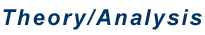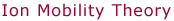Introduction     The ion mobility of a gas-phase ion is the quantity that describes how quickly the ion moves through a drift cell filled with a high pressure of buffer gas under the influence of a weak electric field. Small, compact ions with small collision cross sections drift more quickly than large, extended ions with large collision cross sections. This is similar to the effect that causes an extended paper towel to drift to the ground much more slowly under the influence of gravity and air resistance than a crushed towel of the same mass. Thus, measuring the mobility of an ion yields information about its structure, whether it is compact or extended. Mixtures of compact and extended structures can be separated like in a chromatography experiment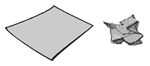(hence "ion chromatography"). However, structural assignments based on mobility results generally require comparison with calculated candidate structures. Those are obtained in our lab by ab initio/density functional theory calculations or molecular modeling using the AMBER force field.     A good reference for studying ion mobility theory is: Mason, E. A.; McDaniel, E. W. Transport Properties of Ions in Gases; Wiley: New York, 1988.     The key instrumental component required to measure an ion's mobility is the drift cell. The details of this component vary depending on the instrument, but in general it is made of copper or glass and has a drift length L of 4-20 cm. It is typically filled with several Torr of helium and the cell temperature can be varied from 80 K to more than 500 K. Guard rings in the cell ensure a uniform electric field E which can be adjusted from approximately 5 to 20 V/cm by changing the voltage V applied across the cell.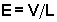A pulse of mass-selected ions is injected into the cell.* The force exerted on them by the electric field is offset by the force of friction caused by collisions of the ions with the buffer gas. As a result, ions drift through the cell with a constant velocity vd that is proportional to the electric field.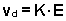In this expression, the proportionality constant K is called the ion mobility. The ion mobility is directly proportional to the buffer gas pressure. It is typically converted to a "reduced" mobility K0 using a value of 760 Torr for P0 and 273.15 K for T0.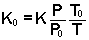By combining the equations above, an expression for the amount of time the ions spend in the drift cell td can be obtained in terms of P/V.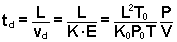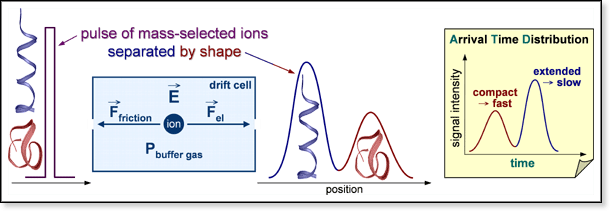The quantity measured experimentally is ion intensity as a function of time, generating a plot called an arrival time distribution (ATD). Arrival time ta is the amount of time between the formation of the initial ion pulse and an ion's arrival at the detector. It is equal to td + t0 where t0 is the amount of time the ions spend outside the drift cell. Plotting ta versus P/V for a given temperature yields a straight line with an intercept equal to t0. The drift time td can be obtained by subtracting t0 from ta. Typical drift times can range anywhere from 100 μs to 10 ms depending on the mobility of the ion and the drift voltage used. The reduced mobility K0 can be obtained from the slope of the line. The Bowers Group uses this type of experiments in several ways (see table below). In general, they involve using the mobility to calculate a collision cross section or using drift times to determine reaction rate constants.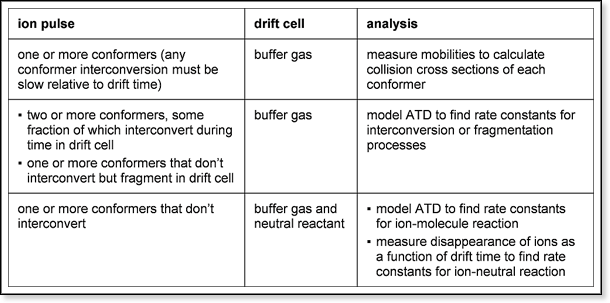Introduction Experimental Collision Cross Sections Kinetics   * All the instruments in the Bowers group have mass-selection capabilities before and after the drift cell except for the ESI instrument, which only has it after the cell.